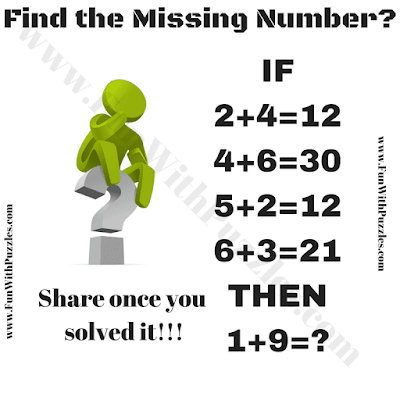This Maths Reasoning Puzzle will test the brain IQ of school-going teens. In this Maths Reasoning Puzzle, you have to decipher the logic of the given Mathematical equations and then solve the last equation to find the missing number which replaces the question mark. So how quickly can you solve this Maths Reasoning Puzzle?Can you solve this reasoning puzzle?

The answer to this "Maths Reasoning Puzzle for Teens", can be viewed by clicking on the button. Please do give your best try before looking at the answer.

Andokan said...

18

Unknown said...

18

Unknown said...

15

Unknown said...

18 that is why
2+4=12 (2×4+4=12)
1+9=18 (1×9+9=18)

E.Yashaswini said...

2*4+4=12
4*6+6=30
5*2+2=12
1*9+9=18